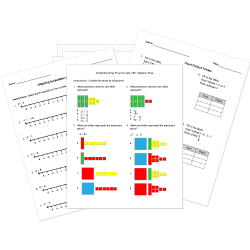Looking for Algebra worksheets?
Check out our pre-made Algebra worksheets!
 Tweet##### Browse Questions
• Arts (311)
• English Language Arts (5352)
• English as a Second Language ESL (3325)
• Health and Medicine (408)
• Life Skills (126)
• Math (1935)

• ### Systems of Equations

• #### Statistics and Probability Concepts

• Physical Education (405)
• Science (4961)
• Social Studies (3723)
• Study Skills and Strategies (19)
• Technology (124)

You can create printable tests and worksheets from these Grade 8 Function and Algebra Concepts questions! Select one or more questions using the checkboxes above each question. Then click the add selected questions to a test button before moving to another page.

Previous Next
Grade 8 Systems of Equations CCSS: 8.EE.C.8, 8.EE.C.8c
Grade 8 Systems of Equations CCSS: 8.EE.C.8, 8.EE.C.8b
Solve the system.
y = 5x − 2
y = −3x + 6
1. (2, 4)
2. (1, 3)
3. (2, 8)
4. (4, 2)
Simplify using a single exponent. $[(-2)^3]^4$
1. $(-2)^7$
2. $-2^12$
3. $2^12$
Simplify the following expression (no negative exponents).
$(3x^6y^3)^3$
1. $6x^9y^6$
2. $9x^9y^9$
3. $9x^18y^9$
4. $27x^18y^9$
In math class there are 16 boys and 12 girls. What is the ratio of girls to total students?
1. $3/4$
2. $3/7$
3. $7/3$
4. $4/3$
$6^15/6^3$
1. $1/6^12$
2. $1/6^18$
3. $6^5$
4. $6^12$
1. $<=$
2. $>=$
3. $=$
4. $>$
1. $4^-2 < 4^-3$
2. $5^-2 > 2 ^ -5$
3. $(-2)^4 = 2^-4$
4. $5^2(5)^-2>2^5(2)^-5$